Home  │ Audio Home Page

Updated July 8, 2012.  See Document History at end for details.

# Floating Source-follower Regulator

One-Bend Amplifier Part 4:  Hum injected in stage 2 of amplifier required the design of a useful general purpose voltage regulator.

### Introduction

At the moment that I realized that the inner feedback loop added to the amplifier for its desirable attributes connected ripple from the VDD1 supply line into open-loop signal path, it became necessary to regulate the offending voltage.  I already held this regulator in my mind as one for non-feedback circuits to get the maximum voltage out that the properties of the regulating MOSFET would allow.

### Regulator Design

The operation of the regulator in figure 1 below is based on a MOSFET being in current amplification mode if the following condition is met.
 (1) VDS > VGS - VT.

With VDS ≈ VGS, regulation is possible if negative ripple deviation does not exceed ≈2VT.

R1 and C1 filter ripple to a level low enough to be eliminated by the diode filter comprised of the parallel diodes and C3.  VG then takes the average of the ripple peaks and dips in VD.  Assuming VG is filtered to a constant value:
 (2) VDS =  VGS ± ½vpeak-ripple

Take the ripple dip as worst case
 (3) VDS =  VGS - ½vpeak-ripple

Combine equations 1 and 3 to get:
 (4) VGS - VT <  VGS - ½vpeak-ripple

Solve to get design equation
 (5) vpeak-ripple  < 2VT.

 Figure 1:  Floating source-follower regulator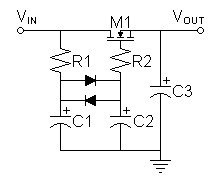Design Methodology
• Calculate capacitance in preceding raw supply to give ripple less than 2VT of regulating MOSFET.
• Calculate R1 for balance between low noise and reasonable current limits.
• Calculate C1 for a RC constant giving ripple low enough at input to diode filter to allow diode impedance high enough to eliminate the remaining ripple.  Initially 25mV seems a good target because it is a figure of merit in the real diode equation.
• Arbitrarily choose C2 to give high RC constant appropriate for expected high Z of parallel diodes, then adjust if necessary during simulation.
• Choose R2 as normal to prevent LC oscillations in regulating MOSFET.
• Calculate C3 to give desired suppression of nonlinearity of regulating MOSFET.
If you want more regulation for peak ripple exceeding two diode drops (≈1.3-1.4V) a clamping diode can be added in parallel with R1 as in figure 2 below.  Then VG will ride at one diode drop above the ripple minimum rather than at the higher ripple average.

 Figure 2:  Floating source-follower regulator clamped to ripple minimum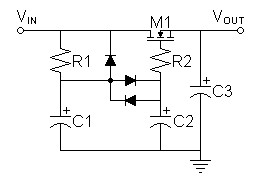The only change in design methodology is the added difficulty in calculating R1 and C1 for ripple reduction to desired small level to drive the diode filter.  Initially, I would estimate the ripple at the junction of R1 and C1 to double due to C1 only seeing half of R1 due to the parallel diode.  Doubling R1 or C1 might be sufficient.

### Power Supply Design

 Figure 3:  Floating source follower regulators added to One-Bend Amplifier power supply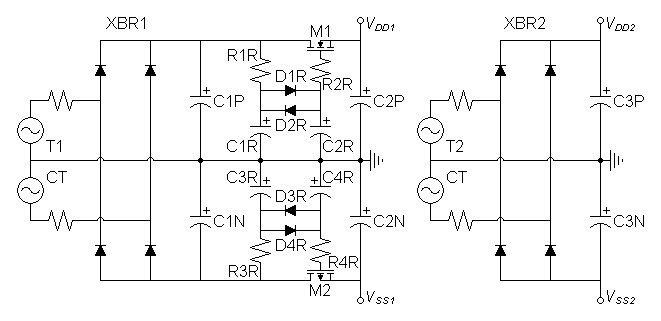SPICE shows IVDD1 = 169mA and IVSS1 = 182mA, assume both could vary with a maximum of 200mA.  Design to 1V ripple at regulator input.
 (6) C1P = i fΔv = 200mA 120Hz × 1V = 1.66667mF
Round up to nearest 20% standard value:  C1P and C1N = 2.2mF
If the raw VDD1 and VSS1 supply are used to supply the regulators for two channels double the value and round up to 4.7mF.

If R1R is chosen to be 1kΩ, calculate C1R to reduce ripple seen at input of diode filter to 25mV.
 (7) fRC = fripple × vfiltered-ripple vripple = 120Hz × 25mV 1V = 3Hz

 (8) C1R = 1 2πfR = 1 2π × 3Hz × 1kΩ = 106.103µF
Round up to nearest 20% standard value:  C1R = 150µF

From datasheet and SPICE model, I estimate the impedance of the filter diodes with a signal of 25mV to be >196MΩ.  Since their operating resistance of filter is so high  choose C2R arbitrarily.
C2R = 10µF

Choose gate resistor
R2R = 100Ω

I used the TF analysis of SPICE to get output impedance representing that of M1 and M2 without effect of C2P and C2N..
ZVDD1 = 2.68632Ω
ZVSS1 = 1.7812Ω

If C2P and C2N are desired to bypass the transfer curves of the regulators M1 and M2, I thought to calculate their values for a pole of 20Hz or lower assuming that the feedback factor in the amplifier will reduce if further at that frequency.  Use worst case of ZVSS1.
 (9) C2N = 1 2πfR = 1 2π × 20Hz × 1.7812Ω = 4.46762mF
Round up to nearest 20% standard value:  C2P and C2N = 4.7mF

Initially transformer T2 was chosen at 48VCT to meet the power specifications.  Since the new design goal was to reduce hum the second transformer could not ride the first piggyback and was separated.  Then the value of T1 was raised to 70VCT after the regulators were verified to allow predrivers to drive output MOSFETs to clipping to as close to VDD2 and VSS2 as possible.

To measure the hum this circuit was designed to reduce do a transient and Fourier analysis at 120Hz and calculate total.

Fourier analysis for vdd1:
No. Harmonics: 10, THD: 71.5238 %, Gridsize: 200, Interpolation Degree: 1

 Harmonic Frequency Magnitude Phase Norm. Mag Norm. Phase 0 0 0 0 0 0 1 120 9.78238e-006 90.3868 1 0 2 240 4.70278e-006 92.1464 0.48074 1.75963 3 360 3.24187e-006 92.5358 0.331399 2.14902 4 480 2.36656e-006 93.605 0.241921 3.21826 5 600 1.93237e-006 94.4977 0.197536 4.11092 6 720 1.58949e-006 95.3495 0.162485 4.96269 7 840 1.37518e-006 96.3245 0.140578 5.93777 8 960 1.19909e-006 97.158 0.122577 6.77119 9 1080 1.06692e-006 98.1152 0.109065 7.72844

Calculate total hum voltage from Fourier data.
 (10) vhum-total = vhum-120Hz × (1 + THD) = 9.78238µV ×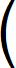1 + 71.5238% 100%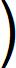= 16.7791µV

Calculate decibel hum relative to 1W level (2.82843V into 8Ω) for relevance to speaker sensitivity
 (11) dBhum = 20 × log16.7791µV 2.82843V= -104.536dB

The calculation for the hum on VSS1 produces nearly identical results.

SPICE Model of standalone power supply setup for hum analysis.

The astute reader might ask why hum had been reduced only modestly after talking about the diode filter eliminating hum.  This expectation only applies to the voltage applied to the gate of the MOSFET.  The hum that results is a factor of an ac drain to source leakage resistance (rds) in the hundreds of kΩ limiting the ability of the MOSFET to completely eliminate the hum in a non-feedback regulator.

### Amplifier Calculations and Simulation Based on New Power Supply

 Figure 4:  One-Bend Amplifier of part 3 used to evaluate new power supply.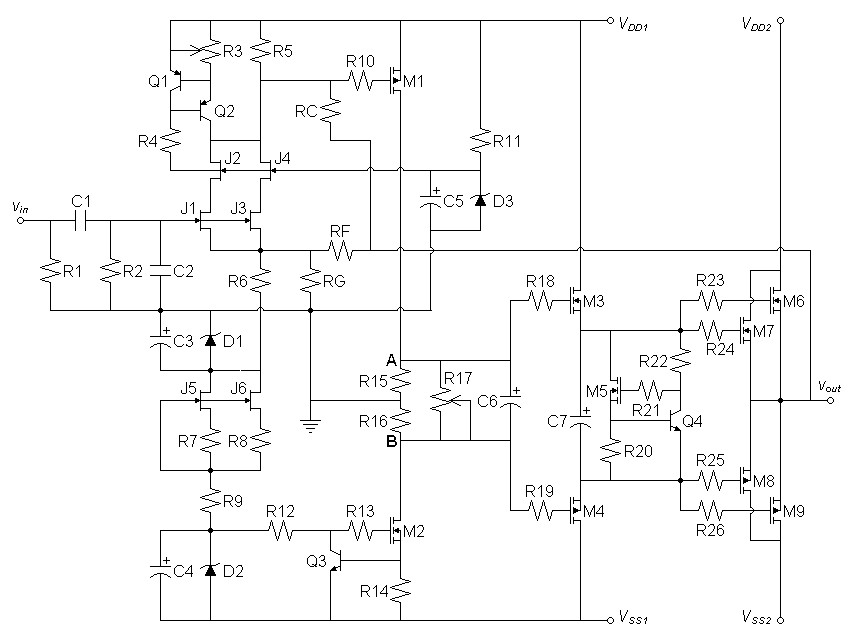Fourier analysis for vout:
No. Harmonics: 16, THD: 73.9737 %, Gridsize: 200, Interpolation Degree: 3

 Harmonic Frequency Magnitude Phase Norm. Mag Norm. Phase 0 0 0 0 0 0 1 120 7.35694e-007 -90.352 1 0 2 240 3.50513e-007 -86.723 0.476439 3.62866 3 360 2.45267e-007 -88.138 0.333382 2.21388 4 480 1.77279e-007 -86.203 0.240968 4.14873 5 600 1.44661e-007 -85.272 0.196632 5.08042 6 720 1.1996e-007 -84.859 0.163057 5.49244 7 840 1.03007e-007 -83.545 0.140014 6.80699 8 960 8.98178e-008 -82.893 0.122086 7.45894 9 1080 8.02879e-008 -81.829 0.109132 8.52283 10 1200 7.23776e-008 -80.99 0.0983799 9.36166 11 1320 6.53764e-008 -80.166 0.0888635 10.1863 12 1440 6.05716e-008 -79.155 0.0823326 11.1972 13 1560 5.54925e-008 -78.23 0.0754288 12.1215 14 1680 5.18678e-008 -77.512 0.0705019 12.8395 15 1800 4.83759e-008 -76.464 0.0657555 13.8882

Calculate total hum voltage from Fourier data.
 (12) vhum-total = vhum-120Hz × (1 + THD) = 735.694nV ×1 + 73.9737% 100%= 1.27991µV

Calculate decibel hum relative to 1W level (2.82843V into 8Ω) for relevance to speaker sensitivity
 (13) dBhum = 20 × log1.27991µV 2.82843V= -126.887dB

This value of hum will not be heard with speakers of any known sensitivity.

A quick look at other SPICE results suggest amplifier performing substantially as in third article except for hum improvement.

SPICE model of amplifier
SPICE model of power supply setup to include in the amplifier model

### Recommended Amplifier Improvement

I recommend that an actual prototype of the amplifier add a coupling capacitor in series with inner feedback resistor RC to give a subsonic zero.  This will improve DC offset at the output in two ways:
• By reducing offset drift with power supply voltage variation.
• By removing DC from inner feedback loop, full DC gain works with global feedback for greatest reduction in DC offset.
I left this capacitor out of the prior simulations because it wrecked the transfer curve analysis as the DC transfer analysis removes capacitors from the circuit and therefore the entire effect of having an inner feedback loop.

For RC of 100kHz and a highpass zero of 1Hz this capacitor calculates:
 (14) CIFB = 1 2πfR = 1 2π × 1Hz × 100kΩ = 1.59155µF
Round up to nearest 20% standard value:  CIFB = 2.2µF